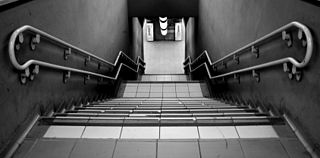# Me and my GiF

Algebra Level 2$\large x \lfloor x \rfloor = 28$

Find the positive value of $x$ such that the equation above is satisfied.

×